## Sunday, November 28, 2010

### Irodov Problem 3.66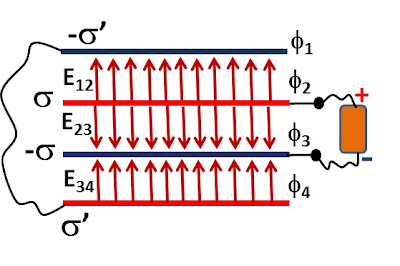(a) Say, plates 2 an 3 are connected across the terminals of a battery as shown. The battery sucks out electrons from plate 2 making it positively charged and deposits them on plate 3 making it negatively charged. Since the total charge has to be conserved, the total charges on plates 2 and 3 must be equal and opposite. Further, all charge within the plates will be uniformly distributed since electrons repel each other and would like to be as far from each other as possible. So let the charges on plates 2 and 3 beand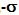respectively.

Since plates 1 and 4 are connected through a conducting wire, electrons are also free to flow between the plates. Consequently the total charge across plates 1 and 4 must also be conserved. Further, plates 1 and 4 must be at the same potential since they are connected by a wire. Hence, we have,Since the potential difference between plates and 2 ans 3 is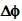, thus,Let us arbitrarily fixFrom (2) and (3) we have,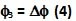Since, the potentials on plates 1 and 4 are the same, this must imply thatand. This means that the direction of electric E12 will be from plate 2 towards plate 1 and that of E34 will be from plate 4 towards plate 3 as shown in the figure.

Now let us put a Gaussian cylinder of area A as shown in Figure 2.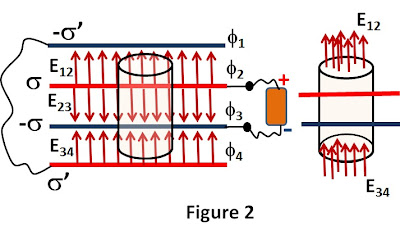The total electric flux through the cylinder will be. The total charge contained in the Gaussian cylinder is. From Gauss law we have,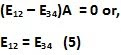The potential at plate 1 will beand that of plate 2 will be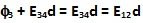. Since both these potentials must be the same (1), we have,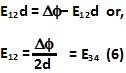Similarly, since the potential between plates 2 and 3 iswe have,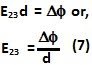(b) The charge densities of plates 1 and 4 will be equal and opposite as the total charge across them is conserved. Let these charges byand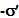respectively. Let us first find the electric field due to a charged plate with surface charge density.Let us consider a Gaussian cylinder across the charged conductor with area A. Let the electric field be E due to the charged conductor. The total electric flux through the cylinder will be 2EA. The total charge contained within this cylinder is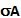. Hence, from Gauss Law we have,For our problem then, the net electric filed is the superposition of the electric fields from all the plates. This is illustrated in Figure 4.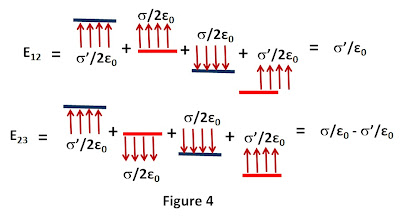As seen from Figure 4,Also we have,